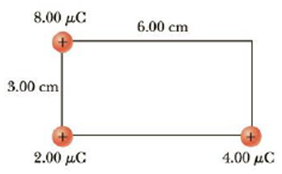Chapter 16, Problem 14P

Chapter
Section
Textbook Problem

Three charges are situated at corners of a rectangle as in Figure P16.13. How much work must an external agent do to move the 8.00-μC charge to infinity?Figure P16.13 Problems 13 and 14.

To determine
The work done to move the charge to infinity.

Explanation

Formula to calculate the work done to move the charge to infinity is,

W=qV

• q is the charge.
• V is the potential.

The potential is given by,

V=keq1r1+keq2r2

• ke is the Coulomb constant.
• q1 , q2 are the charges.
• r1 , r2 are the distances of charges from the point.

From the above equations,

W=qke{q1r1+q2r12+r22}

Substitute 8.00μC for q, 4.00μC for q1 , 2.00μC for q2 , 6

Still sussing out bartleby?

Check out a sample textbook solution.

See a sample solution

The Solution to Your Study Problems

Bartleby provides explanations to thousands of textbook problems written by our experts, many with advanced degrees!

Get Started

Find more solutions based on key concepts